# What is a number line?

In maths, a number line is a visual representation of sequential numbers on a straight, horizontal line. Let’s learn all about this useful tool!Author
Katie WickliffPublished
Sep 25th, 2023# What is a number line?

A number line is a visual representation of sequential numbers on a straight, horizontal line. Let’s learn all about this useful tool!Author
Katie WickliffPublished
Sep 25th, 2023# What is a number line?

In maths, a number line is a visual representation of sequential numbers on a straight, horizontal line. Let’s learn all about this useful tool!Author
Katie WickliffPublished
Sep 25th, 2023Key takeaways

• Number lines boost mental maths skills by bridging concrete and abstract knowledge
• Number lines are used to understand how numbers relate to one another and to solve mathematical problems
• Number lines aren’t just used in the classroom – they’re also found in many real-life places!

## What is a number line?

A number line is a horizontal line with sequential numbers placed at evenly spaced intervals.

While a number line can stretch indefinitely, some have specific starting and ending points. These are called closed number lines. On a number line, the value of the numbers increases as we read from right to left.

### What is a number line graph used for?

A number line, or number line graph, is used to compare the values of different numbers.

Seeing numbers visually on a number line is a great way to help kids develop strong mental maths strategies.

Number lines are also useful for kids moving from concrete representations of numbers (such as blocks and counters) to abstract understanding. This is because they learn that the numbers on a number line get bigger when moving from left to right (or forward) and smaller when moving from right to left (or backward)!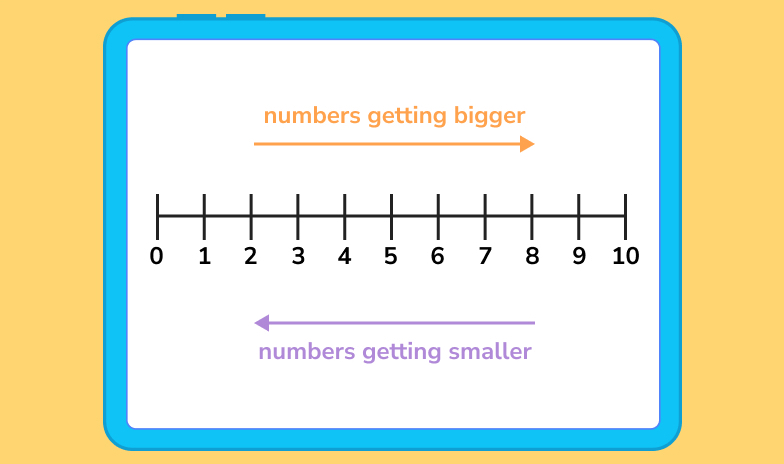Number lines are also used to perform arithmetic operations such as adding, subtracting, multiplying and dividing.

## Explore number lines with DoodleMaths

DoodleMaths is an award-winning maths app that’s filled with games and questions covering the EYFS, KS1 and KS2 curriculum!

Designed to be used for just 10 minutes a day, it creates every child a unique learning experience tailored to their needs, boosting their confidence and ability in maths.

Created by teachers, it automatically targets tricky topics and tops up each child’s knowledge, helping them get ahead in their learning. Why not try it for free today?## Types of number lines

A number line is useful for representing several different types of numbers, including integers, fractions and decimals.

### Integer number line

Integers include positive numbers, negative numbers and zero. Integers are also always whole numbers, which means that fractions and decimals are not considered integers.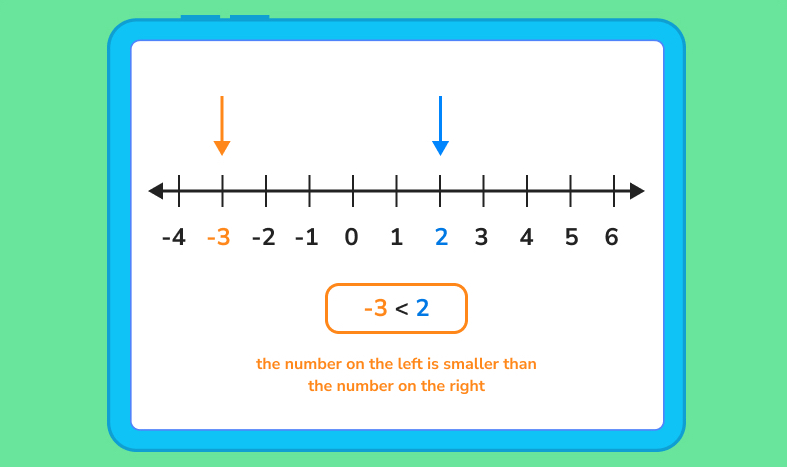The above graphic is an example of a negative and positive number line and is an effective visual aid when kids first learn about negative numbers.

A number line with negatives allows children to see that the positive numbers are to the right of the zero, and the negative numbers are to the left.

### Fractions on a number line

Plotting fractions on a number line lets kids compare fractions and determine their place value.

As we’ve learned, the value of fractions on a number line increases as we move from left to right. Representing fractions on a number line helps children grasp the concept of fractions as part of a whole, as shown in this example: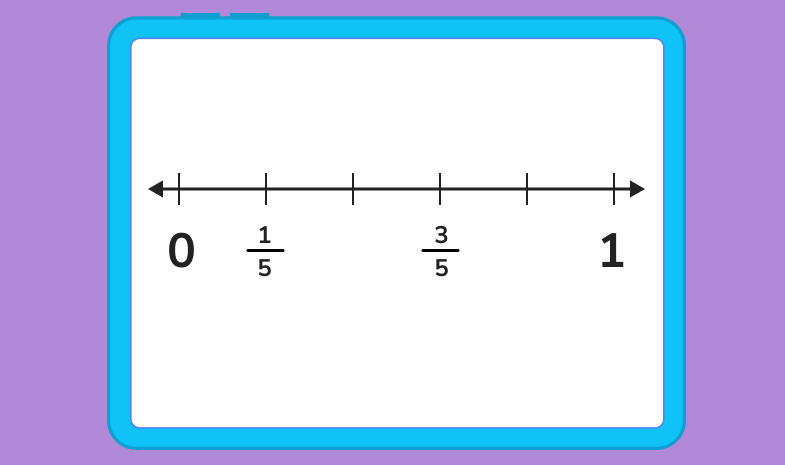### Negative and positive number line: decimal number line

Just like with fractions, a decimal is part of a whole number. A decimal is made up of an integer and a fractional part, and these two parts are separated by a decimal point.

On a decimal number line, the decimals are plotted between two whole numbers and can be both negative and positive. Let’s check out two examples!

Positive decimals on a number line: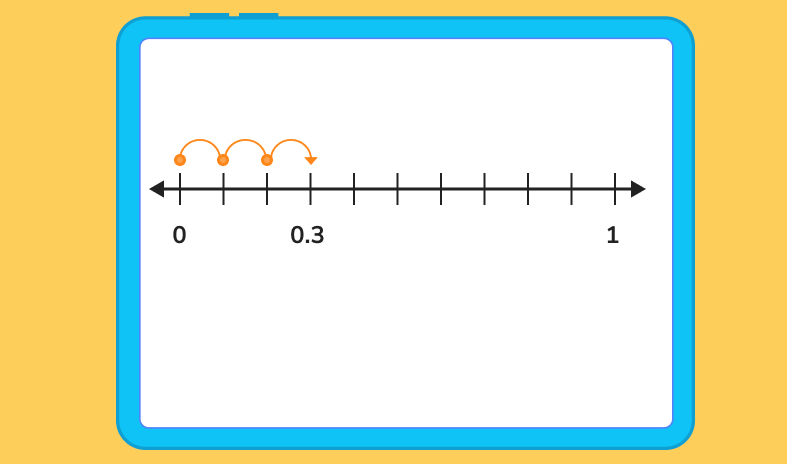Negative decimals on a number line: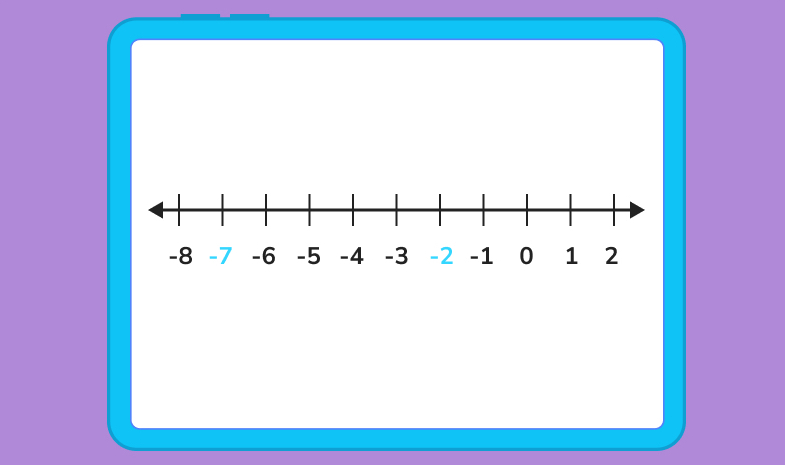### Examples of number lines in real life

Teachers and children aren’t the only ones who use number lines. In fact, our world is full of number lines that serve different purposes! Here are a just a few real life examples:

• Ruler
• Tape measure
• Thermometer
• Pressure gauge
• Long jump event
• Physicians scale## Number line practice questions

Now that we’ve learned about number lines, it’s time to have a go at some questions. Complete the exercises below or head over to DoodleMaths, our award-winning maths app, to build more confidence in using number lines.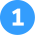Compare the numbers 12 and 17 on a number line. Which is greater?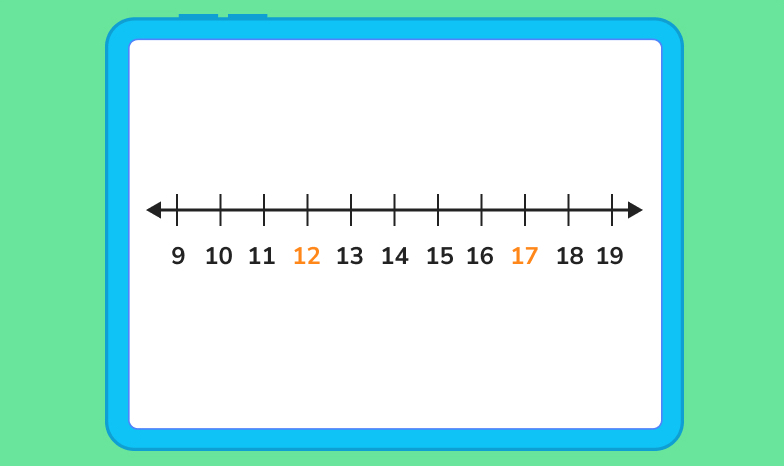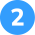Compare the numbers -7 and -2. Which is greater?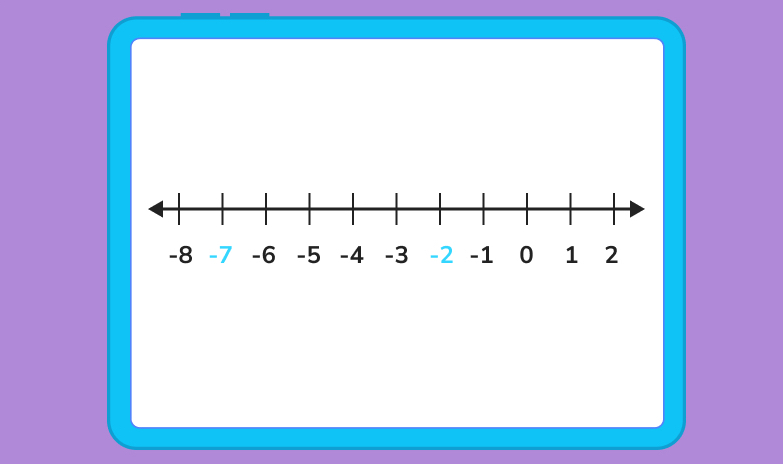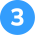Using a number line to 20, solve the addition problem 8+6.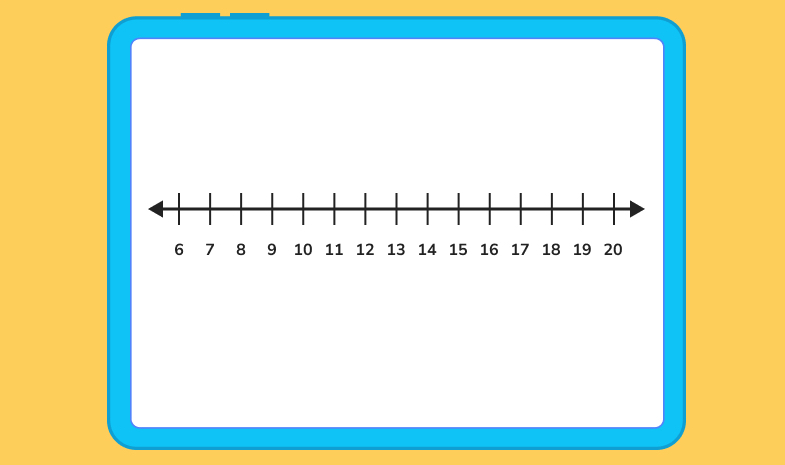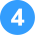Using the number line, solve the subtraction problem 12-7.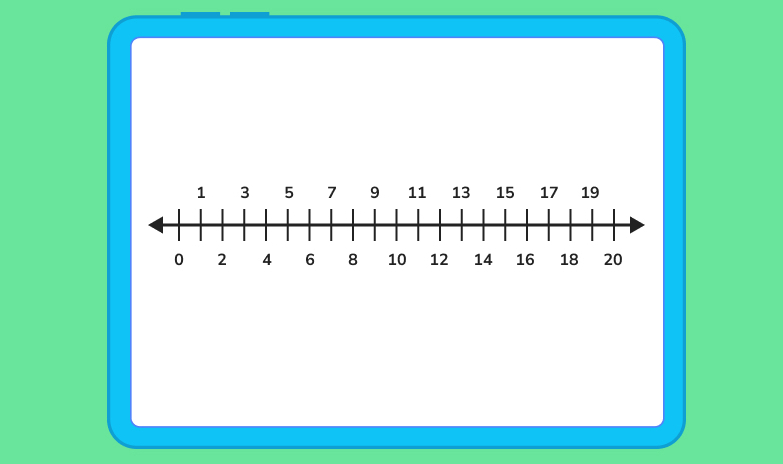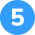Use the number line to multiply 2×8.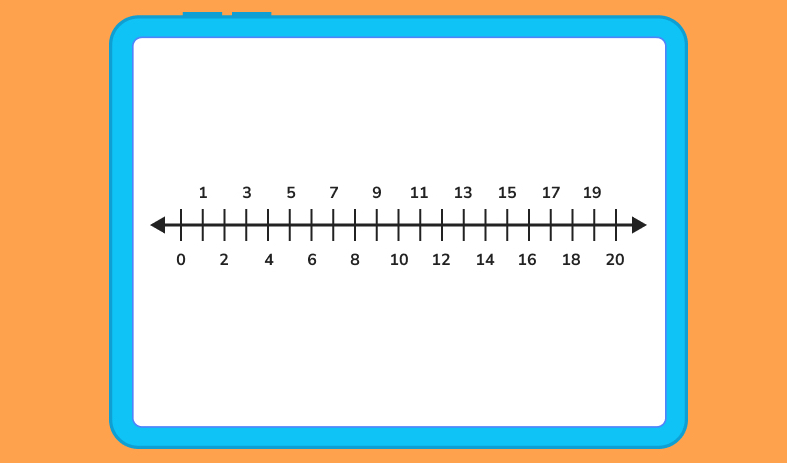The number 12 is to the left of the number 17, so 12 < 17.The number -7 is to the left of the number -2, so -7 < -2.We look at the first number in the problem, which is 8. We then find 8 on the number line and make our mark. Then, we look at the second number in our addition sentence, 6. We count 6 spots to the answer. So, 8 + 6 = 14!We look at the first number in the problem, 12. We find 12 on the number line and make our mark. Then, we look at the second number in our subtraction sentence, 7. We count 7 spots to the left and land on our answer. So, 12 – 7 = 3.To multiply 2×8, we begin at zero and jump 8 spots 2 times. So, 2 x 8 = 16.

In maths, a number line is a visual way to represent numbers in sequential order.

A number line is used to compare numbers and to help people perform mathematical operations, such as addition and subtraction.

One example of a number line in real life is a thermometer.Lesson credits

Katie Wickliff

Katie holds a master’s degree in Education and a bachelor’s degree in both Journalism and English. She has over 15 years of experience as a teacher and is also a certified tutor. Most importantly, Katie is the mother of an 8 and 11-year-old. She's passionate about education and firmly believes that the right tools and support can help every child reach their full potential.

Katie Wickliff

Katie holds a master’s degree in Education and a bachelor’s degree in both Journalism and English. She has over 15 years of experience as a teacher and is also a certified tutor. Most importantly, Katie is the mother of an 8 and 11-year-old. She's passionate about education and firmly believes that the right tools and support can help every child reach their full potential.

## Related posts

Rational and irrational numbers

Learn about the differences between rational and irrational numbers

What are odd and even numbers?

We explore examples of odd and even numbers from 1 to 100

Roman numerals from 1 to 100

Learn the Roman numerals from 1 to 100 and have a go at some questions!

# Are you a parent, teacher or student?

Are you a parent or teacher?

## Hi there!

Book a chat with our team